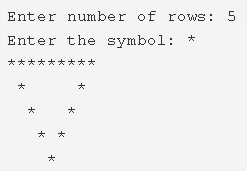# Hollow Inverted Pyramid Star Pattern Program in C

C Program to print a hollow inverted pyramid star pattern – In this article, we will brief in on the various methods to print a hollow inverted pyramid star pattern in C programming.

Suitable examples and sample programs have also been added so that you can understand the whole thing very clearly. The compiler has also been added with which you can execute it yourself.

The ways mentioned in this particular piece are as follows:

• Using For Loop
• Using While Loop
• Using Do-While Loop

As we all know, a pyramid is a three-dimensional figure which has a square as its base and it tapers off into a point giving it the height or the third dimension.

This is what a hollow inverted pyramid looks like:As you can see, you will have to mention the number of rows. Consequently, the pyramid will be printed accordingly taking the number of rows into consideration.

Thus, the various methods used to print a hollow inverted pyramid star pattern in C programming are as follows:

## Using For Loop

1. Read the ‘no.of rows’and read the entered character, store the values into the variables n, ch.

2) The outer for loop iterates through rows from i=n to 1 with the structure for(i=n;i>0;i–).

3) The 1st inner for loop iterates through columns with the structure for(j=1;j<=n-i;j++).

It prints space.

4) For i=1 or n

The 2nd inner for loop iterates through columns with the structure for(j=1;j<=i*2-1;j++)

Prints symbol.

5) For i!=1 or i!=n

The 3rd inner for loop iterates through columns with the structure for(j=1;j<=i*2-1;j++)

Prints symbol if j=1 or j=i*2-1.Otherwise it prints space.

6) Cursor comes to nextline for each iteration of i.

Output:

### Using While Loop

1. The while loop checks the condition first, if the condition is true then it executes the code once. If the condition is false then it terminates the loop.

2) i=n, The outer while loop iterates through rows until the condition i>0 becomes false.

3) j=1, The 1st inner while loop iterates through columns until the condition j<=n-i becomes false.

It prints space.

j value increased by 1. The 1st inner loop again checks the condition.

4) For 1st row, last row

The 2nd inner while loop iterates through columns until the condition j<=i*2-1 becomes false.

It prints symbol.j value increased by 1. The 2nd inner loop again checks the condition.

5) For other than the 1st row, last row

The 3rd inner while loop iterates through columns until the condition j<=i*2-1 is false.

It prints symbol if j=1 or j=(i*2)-1. If the condition is false then it prints space.

j value increased by 1. The 3rd inner loop again checks the condition.

6) Cursor comes to next line, i value decreased by 1. The outer while loop again checks the condition.

Output:

#### Using Do-While Loop

1. The do-while loop executes the code one time then checks the condition. If the condition is true then loop iterates again.

2) i=n, The outer do-while loop iterates through rows.

3) j=1, The 1st inner do-while loop iterates through columns.

Prints space. j value increased by 1.

Checks the condition j<=n-i+1. If the condition is true then the loop iterates again. Repeats until the condition becomes false.

4) For i=1 or n

The 2nd inner do-while loop iterates through columns.

Prints symbol. j value increased by 1.

Checks the condition j<=i*2-1.If the condition is true then the loop iterates again. Repeats until the condition becomes false.

5) For i!=1 or i!=n

The 3rd inner do-while loop iterates through columns.

If j=1 or j=i*2-1 it prints symbol. Otherwise it prints space

j value increased by 1.

Checks the condition j<=i*2-1. If the condition is true then the loop iterates again. Repeats until the condition is false.

6) Cursor comes to next line.i value decreased by 1.

7) Checks the condition i>0. If the condition is true then outer loop iterates again. Repeats until the condition fails.

Output:
x

## C Program To Print Number Of Days In A Month | Java Tutoring

C program to input the month number and print the number of days in that ...